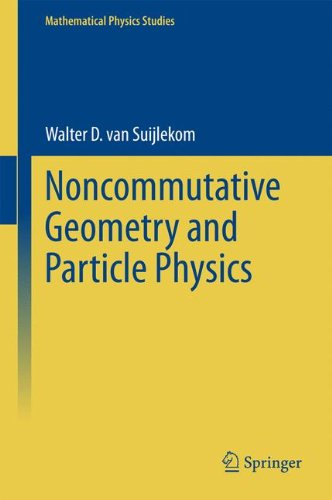# Perturbations of the metric and Weyl’s Eichinvarianz

Thanks to David Broadhurst for stressing the following point during my lecture at a summer school in Les Houches.

Whilst introducing gauge fields from noncommutative spin manifolds (aka spectral triples) I first explained how the Dirac operator can be seen as a metric on a (possibly noncommutative) space described via Connes’ distance formula. Then the action of a unitary in the algebra of coordinates was given as a gauge transformation on the Dirac operator, generating a pure gauge field.

What David noticed was that this is in compelling agreement with Weyl’s old idea of gauge invariance. Indeed, the term Eichinvarianz was preceded by Maβstabinvarianz in the original work (see Yang’s review below). This is precisely the notion captured by noncommutative geometry: a gauge transformation actually acts on the metric (the Dirac operator) but leaves the distance function invariant.

# Announcement book “Noncommutative Geometry and Particle Physics” by Walter van Suijlekom

My book “Noncommutative Geometry and Particle Physics”  is due to appear this summer with Springer:

This textbook provides an introduction to noncommutative geometry and presents a number of its recent applications to particle physics. It is intended for graduate students in mathematics/theoretical physics who are new to the field of noncommutative geometry, as well as for researchers in mathematics/theoretical physics with an interest in the physical applications of noncommutative geometry. In the first part, we introduce the main concepts and techniques by studying finite noncommutative spaces, providing a “light” approach to noncommutative geometry. We then proceed with the general framework by defining and analyzing noncommutative spin manifolds and deriving some main results on them, such as the local index formula. In the second part, we show how noncommutative spin manifolds naturally give rise to gauge theories, applying this principle to specific examples. We subsequently geometrically derive abelian and non-abelian Yang-Mills gauge theories, and eventually the full Standard Model of particle physics, and conclude by explaining how noncommutative geometry might indicate how to proceed beyond the Standard Model.

# Re-launch of noncommutativegeometry.nl

After a transition period it is time to re-launch this website. It has been transformed from a website dedicated to the workshop at the Lorentz Center in Leiden in October 2013 to a more general repository and discussion platform for noncommutative geometry and its applications to particle physics. Everyone is welcome to share his or her thoughts here.

For now, let me mention some good video excerpts from a lecture that Alain Connes gave in Nijmegen, see the documents section for that.

Also, allow me to advertise my upcoming book “Noncommutative Geometry and Particle Physics” which is due to appear this summer with Springer:This textbook provides an introduction to noncommutative geometry and presents a number of its recent applications to particle physics. It is intended for graduate students in mathematics/theoretical physics who are new to the field of noncommutative geometry, as well as for researchers in mathematics/theoretical physics with an interest in the physical applications of noncommutative geometry. In the first part, we introduce the main concepts and techniques by studying finite noncommutative spaces, providing a “light” approach to noncommutative geometry. We then proceed with the general framework by defining and analyzing noncommutative spin manifolds and deriving some main results on them, such as the local index formula. In the second part, we show how noncommutative spin manifolds naturally give rise to gauge theories, applying this principle to specific examples. We subsequently geometrically derive abelian and non-abelian Yang-Mills gauge theories, and eventually the full Standard Model of particle physics, and conclude by explaining how noncommutative geometry might indicate how to proceed beyond the Standard Model.